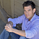4936 lượt xem
4936
study("HullMA",overlay=true)
n=input(title="period",type=integer,defval=16)

n2ma=2*wma(close,round(n/2))
nma=wma(close,n)
diff=n2ma-nma
sqn=round(sqrt(n))

n2ma1=2*wma(close,round(n/2))
nma1=wma(close,n)
diff1=n2ma1-nma1
sqn1=round(sqrt(n))

n1=wma(diff,sqn)
n2=wma(diff1,sqn)
c=n1>n2?green:red
ma=plot(n1,color=c)Hey! Why does the hull ma change from red to green back to red. What moving average crosses are they to have them switch colors? I am trying to code a bot that buys when the hullma is green and sells when the hullma is red, and was wondering which moving averages cause them to change colors. Thanks!
Phản hồigreat indicator. Thank you. Question: If I wanted to add a LONG alert condition to an existing script in which the alert fires ONLY IF the Candle Opening price is BELOW the HMA, or ONLY if the HMA is showing GREEN, do you have any guidance for this? I'm new to coding and can't seem to figure it out
Phản hồijamjan33
@jamjan33, Did you ever get an answer for this? I really really like the single color changing line but cant seem to find anything similar for my MT4 chart.....
Phản hồi